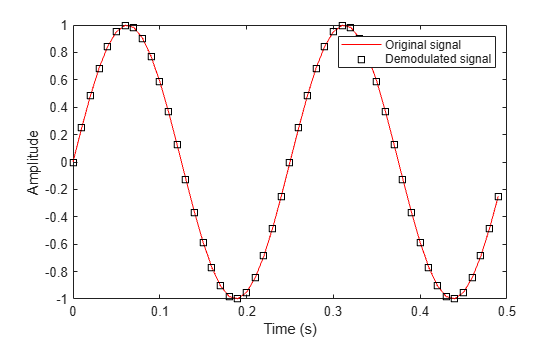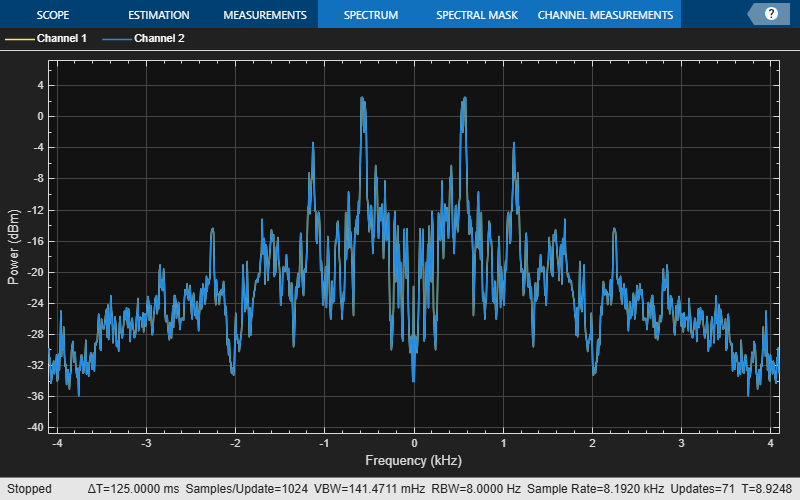# comm.FMDemodulator

Demodulate using FM method

## Description

The `FMDemodulator` System object™ demodulates an FM modulated signal.

To FM demodulate a signal:

1. Define and set up the `FMDemodulator` object. See Construction.

2. Call `step` to FM demodulate a signal according to the properties of `comm.FMDemodulator`. The behavior of `step` is specific to each object in the toolbox.

Note

Starting in R2016b, instead of using the `step` method to perform the operation defined by the System object™, you can call the object with arguments, as if it were a function. For example, ```y = step(obj,x)``` and `y = obj(x)` perform equivalent operations.

## Construction

`H = comm.FMDemodulator` creates a demodulator System object, `H`, that frequency demodulates an input signal.

`H = comm.FMDemodulator(mod)` creates an FM demodulator object whose properties are determined by the corresponding FM modulator object, `mod`.

`H = comm.FMDemodulator(Name,Value)` creates an FM demodulator object with each specified property `Name` set to the specified `Value`. `Name` must appear inside single quotes. You can specify additional name-value pair arguments in any order as (`Name1`,`Value1`,...,`NameN`,`ValueN`).

## Properties

 `FrequencyDeviation` Peak deviation of the output signal frequency (Hz) Specify the frequency deviation of the FM demodulator in Hz as a positive real scalar. The default value is `75e3`. The system bandwidth is equal to twice the sum of the frequency deviation and the message bandwidth. This property is nontunable. `SampleRate` Sample rate of input signal (Hz) Specify the sample rate in Hz as a positive real scalar. The default value is `240e3`. The output sample rate is equal to the input sample rate. This property is nontunable.

## Methods

 reset Reset states of the FM demodulator object step Applies FM baseband demodulation
Common to All System Objects
`release`

Allow System object property value changes

## Examples

collapse all

Modulate and demodulate a sinusoidal signal. Plot the demodulated signal and compare it to the original signal.

Set the example parameters.

```fs = 100; % Sample rate (Hz) ts = 1/fs; % Sample period (s) fd = 25; % Frequency deviation (Hz)```

Create a sinusoidal input signal with duration 0.5 s and frequency 4 Hz.

```t = (0:ts:0.5-ts)'; x = sin(2*pi*4*t);```

Create FM modulator and demodulator System objects.

```fmmod = comm.FMModulator('SampleRate',fs,'FrequencyDeviation',fd); fmdemod = comm.FMDemodulator('SampleRate',fs,'FrequencyDeviation',fd);```

FM modulate the input signal and plot its real part. You can see that the frequency of the modulated signal changes with the amplitude of the input signal.

```y = fmmod(x); plot(t,[x real(y)])```Demodulate the FM modulated signal.

`z = fmdemod(y);`

Plot the input and demodulated signals. The demodulator output signal exactly aligns with the input signal.

```plot(t,x,'r',t,z,'ks') legend('Input Signal','Demod Signal') xlabel('Time (s)') ylabel('Amplitude')```Create an FM demodulator System object? from an FM modulator object. Modulate and demodulate audio data loaded from a file and compare its spectrum with that of the input data.

Set the example parameters.

```fd = 50e3; % Frequency deviation (Hz) fs = 300e3; % Sample rate (Hz) ```

Create an FM modulator System object.

```MOD = comm.FMModulator('FrequencyDeviation',fd,'SampleRate',fs); ```

Create a companion demodulator object based on the modulator.

```DEMOD = comm.FMDemodulator(MOD); ```

Verify that the properties are identical in the two System objects.

```MOD DEMOD ```
```MOD = comm.FMModulator with properties: SampleRate: 300000 FrequencyDeviation: 50000 DEMOD = comm.FMDemodulator with properties: SampleRate: 300000 FrequencyDeviation: 50000 ```

Load audio data into structure variable, `S`.

```S = load('handel.mat'); data = S.y; fsamp = S.Fs; ```

Create a spectrum analyzer System object.

```SA = dsp.SpectrumAnalyzer('SampleRate',fsamp,'ShowLegend',true); ```

FM modulate and demodulate the audio data.

```modData = step(MOD,data); demodData = step(DEMOD,modData); ```

Verify that the spectrum plot of the input data (Channel 1) is aligned with that of the demodulated data (Channel 2).

```step(SA,[data demodData]) ```Playback an audio file after applying FM modulation and demodulation. The example takes advantage of the characteristics of System objects™ to process the data in streaming mode.

Load the audio file, `guitartune.wav`, using an audio file reader object.

```AUDIO = dsp.AudioFileReader... ('guitartune.wav','SamplesPerFrame',4410);```

Create an audio device writer object for audio playback.

`AUDIOPLAYER = audioDeviceWriter;`

Create modulator and demodulator objects having default properties.

```MOD = comm.FMModulator; DEMOD = comm.FMDemodulator;```

Read audio data, FM modulate, FM demodulate, and playback the demodulated signal, `z`.

```while ~isDone(AUDIO) x = step(AUDIO); % Read audio data y = step(MOD,x); % FM modulate z = step(DEMOD,y); % FM demodulate step(AUDIOPLAYER,z); % Playback the demodulated signal end```

Modulate and demodulate a sinusoidal signal. Plot the demodulated signal and compare it to the original signal.

Set the example parameters.

```fs = 100; % Sample rate (Hz) ts = 1/fs; % Sample period (s) fd = 25; % Frequency deviation (Hz)```

Create a sinusoidal input signal with duration 0.5 s and frequency 4 Hz.

```t = (0:ts:0.5-ts)'; x = sin(2*pi*4*t);```

Create FM modulator and demodulator System objects.

```fmmod = comm.FMModulator('SampleRate',fs,'FrequencyDeviation',fd); fmdemod = comm.FMDemodulator('SampleRate',fs,'FrequencyDeviation',fd);```

FM modulate the input signal and plot its real part. You can see that the frequency of the modulated signal changes with the amplitude of the input signal.

```y = fmmod(x); plot(t,[x real(y)])```Demodulate the FM modulated signal.

`z = fmdemod(y);`

Plot the input and demodulated signals. The demodulator output signal exactly aligns with the input signal.

```plot(t,x,'r',t,z,'ks') legend('Input Signal','Demod Signal') xlabel('Time (s)') ylabel('Amplitude')```## Selected Bibliography

 Chakrabarti, I. H., and Hatai, I. “A New High-Performance Digital FM Modulator and Demodulator for Software-Defined Radio and Its FPGA Implementation.” International Journal of Reconfigurable Computing. Vol. 2011, No. 10.1155/2011, 2011, p. 10.

 Taub, Herbert, and Donald L. Schilling. Principles of Communication Systems. New York: McGraw-Hill, 1971, pp. 142–155.

## Algorithms

Represent a frequency modulated passband signal, Y(t), as

`$Y\left(t\right)=A\mathrm{cos}\left(2\pi {f}_{c}t+2\pi {f}_{\Delta }{\int }_{0}^{t}x\left(\tau \right)d\tau \right)\text{\hspace{0.17em}},$`

where A is the carrier amplitude, fc is the carrier frequency, x(τ) is the baseband input signal, and fΔ is the frequency deviation in Hz. The frequency deviation is the maximum shift from fc in one direction, assuming |x(t)| ≤ 1.

A baseband FM signal can be derived from the passband representation by downconverting it by fc such that

`$\begin{array}{c}{y}_{s}\left(t\right)=Y\left(t\right){e}^{-j2\pi {f}_{c}t}=\frac{A}{2}\left[{e}^{j\left(2\pi {f}_{c}t+2\pi {f}_{\Delta }{\int }_{0}^{t}x\left(\tau \right)d\tau \right)}+{e}^{-j\left(2\pi {f}_{c}t+2\pi {f}_{\Delta }{\int }_{0}^{t}x\left(\tau \right)d\tau \right)}\right]{e}^{-j2\pi {f}_{c}t}\\ =\frac{A}{2}\left[{e}^{j2\pi {f}_{\Delta }{\int }_{0}^{t}x\left(\tau \right)d\tau }+{e}^{-j4\pi {f}_{c}t-j2\pi {f}_{\Delta }{\int }_{0}^{t}x\left(\tau \right)d\tau }\right]\text{\hspace{0.17em}}.\end{array}$`

Removing the component at -2fc from ys(t) leaves the baseband signal representation, y(t), which is expressed as

`$y\left(t\right)=\frac{A}{2}{e}^{j2\pi {f}_{\Delta }{\int }_{0}^{t}x\left(\tau \right)d\tau }.$`

The expression for y(t) is rewritten as

`$y\left(t\right)=\frac{A}{2}{e}^{j\varphi \left(t\right)}\text{\hspace{0.17em}},$`

where $\varphi \left(t\right)=2\pi {f}_{\Delta }{\int }_{0}^{t}x\left(\tau \right)d\tau$, which implies that the input signal is a scaled version of the derivative of the phase, ϕ(t).

A baseband delay demodulator is used to recover the input signal from y(t).A delayed and conjugated copy of the received signal is subtracted from the signal itself,

`$w\left(t\right)=\frac{{A}^{2}}{4}{e}^{j\varphi \left(t\right)}{e}^{-j\varphi \left(t-T\right)}=\frac{{A}^{2}}{4}{e}^{j\left[\varphi \left(t\right)-\varphi \left(t-T\right)\right]}\text{\hspace{0.17em}},$`

where T is the sample period. In discrete terms, wn=w(nT), and

`$\begin{array}{l}{w}_{n}=\frac{{A}^{2}}{4}{e}^{j\left[{\varphi }_{n}-{\varphi }_{n-1}\right]}\text{\hspace{0.17em}}\text{,}\\ {v}_{n}={\varphi }_{n}-{\varphi }_{n-1}\text{\hspace{0.17em}}.\end{array}$`

The signal vn is the approximate derivative of ϕn, such that vnxn.